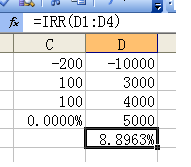# 程序设计能力实训

1110. 计算内部回报率Excel 计算结果 $r$ 大约为 $8.8963\%$

### 输入格式

$1$ 行：整数 $T$ ($1 \le T \le 10$) 为问题数。

$2$~$3$ 行：第一个问题的数据。一行整数 $n$ ($1 \le n \le 100$) 表示项目生产年数。后面 1 行包含 $n+1$ 个由一个空格分隔的整数，表示投入的建设资金 $c_0$$n$ 年中每年的利润 $c_i$$c_0>0,0 \le c_i \le 1 000 000 000$

$4$~$2T+1$ 行：后面问题的数据，格式与第一个问题相同。

### 样例

Input
3
2
200 100 100
3
10000 3000 4000 5000
5
3000 100 100 100 100 100

Output
case #0:
0.0000%
case #1:
8.8963%
case #2:
-40.1791%